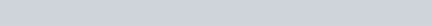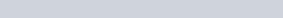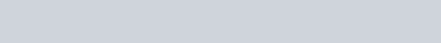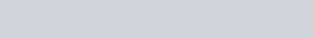Livio/ June 30, 2018/ Excel, Power Query/ 0 comments

## Create a Fixed Length CSV with Power Query

The can make your life very easy when it comes to turn a table into a fixed length CSV file. The ones I will use in this post are CombineTextByRanges and CombineTextByLengthsCombineTextByRanges is simply a more advanced version of CombineTextByLengths.  Fixed Length CSV with Power Query

## The Source Table

Imagine to start off with a source table like the one below and you need to convert it to a csv or text file where the first column has a fixed length of 10 characters, the second column a fixed length of 100 characters, the 3rd column a fixed length of 10 characters and so does the 4th column.## Load the table into Power Query

I have named this table “Data”, in order to import it into Power Query, the M code is:The next step is to convert the data type of each column into type text:And then convert the table into a list by using the Table.ToRows function, which converts the table into a list where each list item is a list of the values of each recordSo far, nothing special. The next step is to create the function. This function accepts two arguments. The first argument is a list consisting of numbers which represent the lengths you want to give to each element of the list we previous created, RowsList. This means that if you want to create a text string from a list of 4 elements where the first element has a length of 10, the second element has a length of 100, the 3rd element has a length of 10 and the 4th element has a length of 10, you would pass to this function as first argument {10,100,10,10}. The elements within the list that have a length higher than the one specified will be truncated. The second argument is a template on which the string will be typed, normally this argument equals a string of the same repeated character which has a length equal to the sum of the arguments passed as first argument, it is useful because by default the function will use blank spaces but by passing another character you can use this character to divide the words.

The Combiner.CombineTextByRanges function behaves similarly to the one previously described, the difference between the two is that the first argument passed to CombineTextByRanges  is a list of lists. Each list element has two elements, the first one specifying, based on a 0 based count, the position on which the first element will be written and the second specifying the length of the first element. Taking our example, if we want Sec ID to start at position 0 and be of 10 in length, Sec Name to start at position 15 and be of 100 in length, Sec Price to start at position 120 and be of 10 in length and Sec Notional to start as position 140 and be of 10 in length, this argument would be {{0,10},{15,100},{120,10},{140,10}}. The second argument behaves like the one in CombineTextByLengths.

After this little explanation, we can go ahead and create our CombineTextByLengths function:And apply the transformation to our RowsList:   Fixed Length CSV with Power QueryThe result is (it is very important that you check the option Monospaced) (Click the image to enlarge)Now you are left with a list where each element has been combined based on your combiner function. If you want to go the extra mile to turn this list into a text string joined by a line feed, you can simply do:Full Code:Must read books if you want to become a Power Query guru: